# Introduction To Quadratics And Their Graphs

The most basic quadratic function is   $$f\left( x \right) = {x^2}$$ , whose graph is shown in Fig - 1.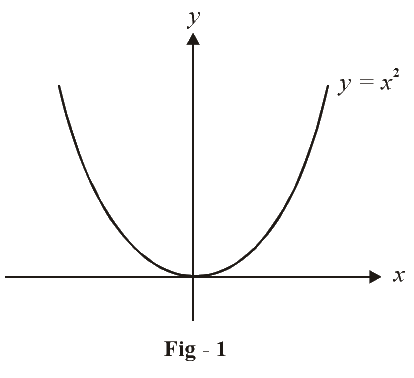All other quadratic expressions can be written in terms of scaled and shifted versions of this basic function.This is what we did in the unit on functions. We are repeating it again here for the sake of a comprehensive discussion.

The general quadratic expression can be written as

$f\left( x \right) = a{x^2} + bx + c a \ne 0$

Our purpose here is to draw the graph of this function and find its roots. By the roots of a function $$f\left( x \right)$$ , we mean those values of x for which $$f\left( x \right)$$ becomes 0.

Graphically, roots correspond to those values of x at which the graph of $$f\left( x \right)$$ crosses the x-axis (becomes 0).

It might be possible that the graph does not cross the x-axis at all, or $$f\left( x \right)$$ never becomes 0. In this case we say that $$f\left( x \right)$$ has non-real (complex) roots.

Now, to do something useful with the expression $$a{x^2} + bx + c,$$ we rearrange it as follows:

\begin{align}&f\left( x \right) = a{x^2} + bx + c\\\,\,\,\,\,\,\,\,\,\,\, &\qquad= a\left( {{x^2} + \frac{{bx}}{a} + \frac{c}{a}} \right)\\\end{align}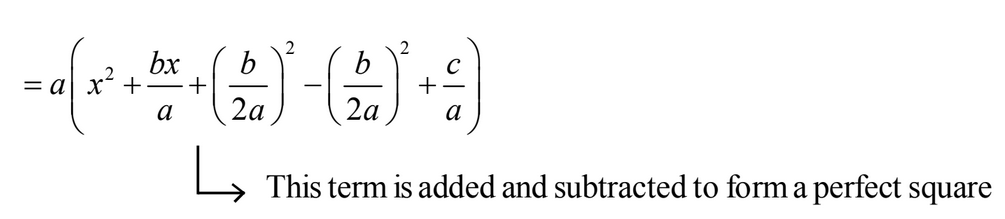\begin{align}& = a\left\{ {{{\left( {x + \frac{b}{{2a}}} \right)}^2} - \frac{{{b^2}}}{{4{a^2}}} + \frac{a}{c}} \right\}\\ &= a{\left( {x + \frac{b}{{2a}}} \right)^2} - \frac{{\left( {{b^2} - 4ac} \right)}}{{4a}}\\ &= a{\left( {x + \frac{b}{{2a}}} \right)^2} - \frac{D}{{4a}}\qquad\qquad\qquad\qquad\dots{\rm{ (1)}}\\ \end{align}

Where $$D = {b^2} - 4ac$$ is called the discriminant of the quadratic expression. The rearranged expression gives useful information as follows:

(A)       $$\fbox{ The roots of f ( x)}$$

To find the roots we simply put

\begin{align}&\,\,\,\,\,\,\,\,\,\,\,\,\,\,\,\,f\left( x \right) = a{\left( {x + \frac{b}{{2a}}} \right)^2} - \frac{D}{{4a}} = 0\\ &\quad\Rightarrow a{\left( {x + \frac{b}{{2a}}} \right)^2} = \frac{D}{{4a}}\\ &\quad\Rightarrow x + \frac{b}{{2a}} = \pm \frac{{\sqrt D }}{{2a}}\\ &\quad\Rightarrow x = \frac{{ - b \pm \sqrt D }}{{2a}}\end{align}

Thus, we see that there are two roots of $$f\left( x \right):$$

${x_1} = \frac{{ - b - \sqrt D }}{{2a}},\,\,{x_2} = \frac{{ - b + \sqrt D }}{{2a}}\,\,\,\,\,\,\,\,\,\,\,\,\,\,\,\,\,\,\,\,\,\,\,\,\,\,\,\,\,\,...{\rm{ (2)}}$

(B)       $$\fbox{ The graph of f ( x)}$$

In terms of scaling and shifting operations, the graph of $$f\left( x \right)$$ can be obtained in the following steps:

\begin{align}{x^2}\xrightarrow{{Scaling}}a{x^2}\xrightarrow[{Shifting}]{{Horizontal}}a{\left( {x + \frac{b}{{2a}}} \right)^2}\xrightarrow[{Shifting}]{{Vertical}}a{\left( {x + \frac{b}{{2a}}} \right)^2} - \frac{D}{{4a}}\end{align}

* If a > 0, the graph is an upwards parabola else it opens downwards.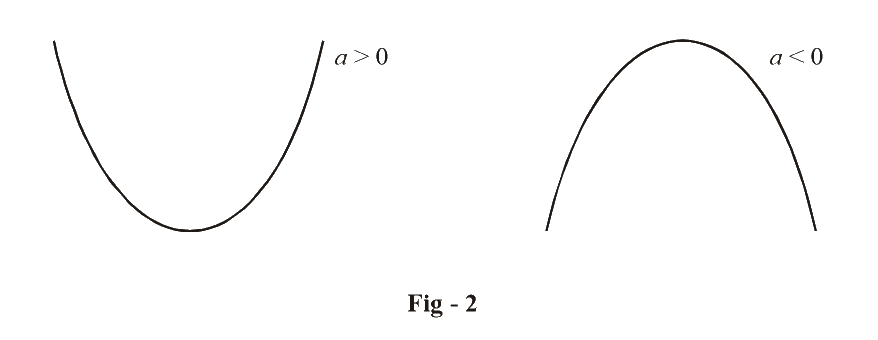* If \begin{align}\frac{b}{{2a}} > 0,\end{align} the parabola is shifted left else if \begin{align}\frac{b}{{2a}} < 0,\end{align} it gets right shifted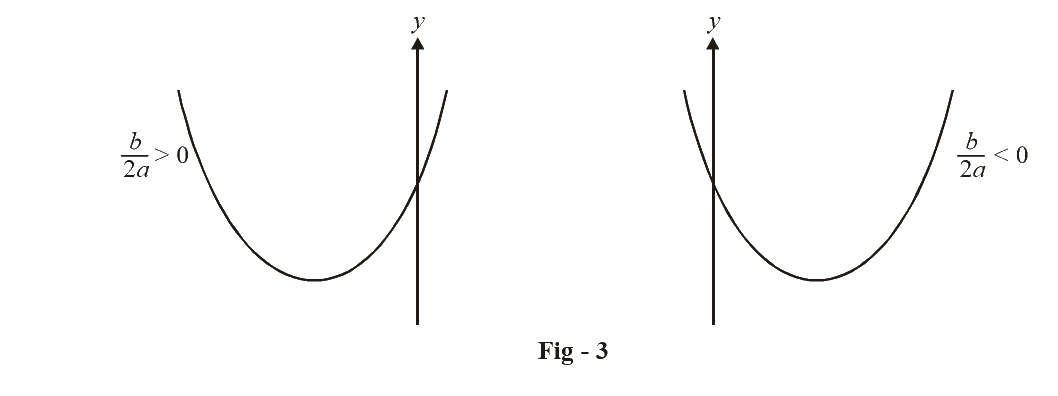* If \begin{align}\frac{D}{{4a}} > 0,\end{align} the graph gets shifted downwards else if  it \begin{align}\frac{D}{{4a}} > 0,\end{align}  gets shifted upwards.

The figure below is an example of this for $$a > 0$$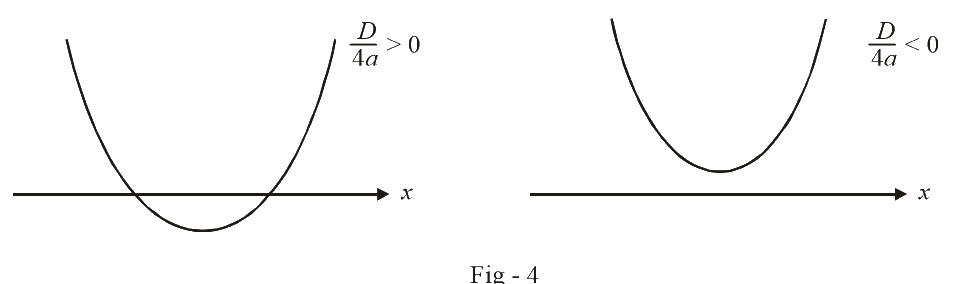* The co-ordinates of the vertex of the parabola are given by \begin{align}\left( {\frac{{ - b}}{{2a}},\frac{{ - D}}{{4a}}} \right)\end{align}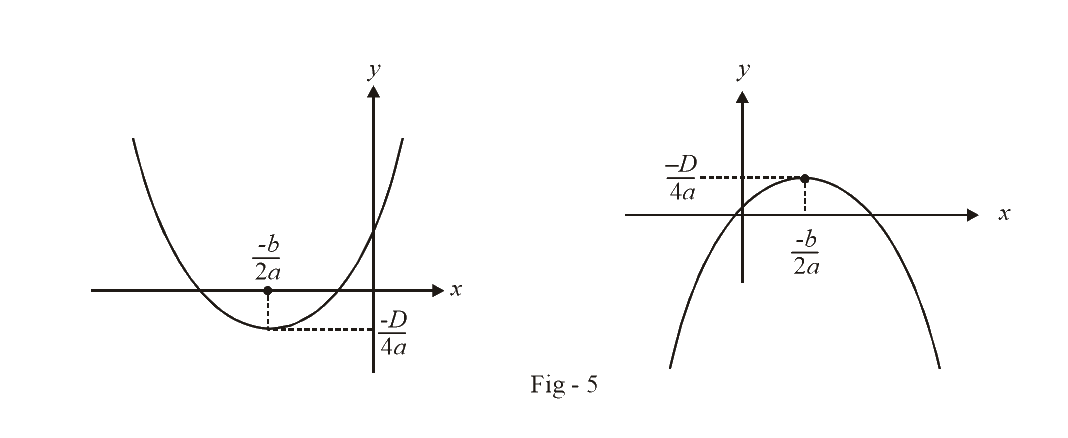* If the parabola cuts the x-axis at two distinct points, we say that $$f\left( x \right)$$ has two distinct roots given by (2). If the parabola just touches the x-axis (at a single point), or in other words the vertex of the parabola lies on the x-axis, we say that $$f\left( x \right)$$ has real and equal roots (both roots are the same). This is obvious from (2), since if the vertex lies on the x-axis, D = 0 so that

${x_1} = {x_2} = \frac{{ - b}}{{2a}}$

If the parabola does not cross the x-axis at all, we say that $$f\left( x \right)$$ has non-real (or imaginary roots). Again this is obvious from the formula for the roots, (2). If the parabola lies entirely above the x-axis, the y-coordinate of the vertex $$\left( { - D/4a} \right)$$ is positive and a is also positive, implying D is negative. Similarly if the parabola lies entirely below the x-axis, $$\left( { - D/4a} \right)$$ is negative and a is also negative, implying D is again negative. Thus, the formula for the roots gives complex values of x.

The graph in the figure below illustrate these three cases for $$a > 0$$  and $$a > 0$$ separately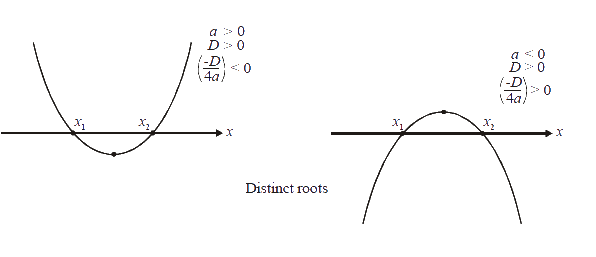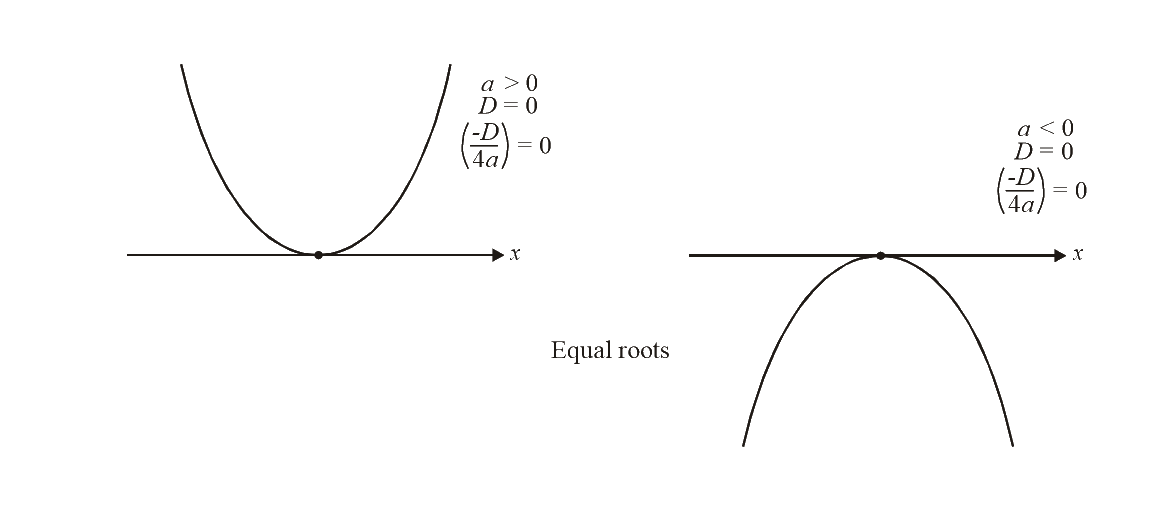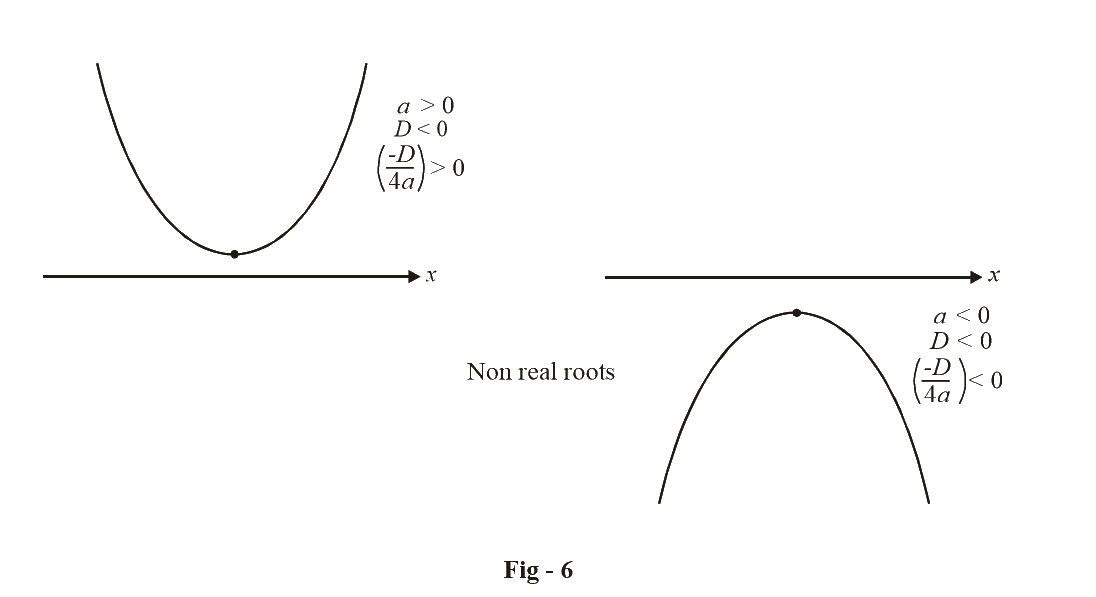We see that for any a:

$\begin{array}{l}D > 0 \; \Rightarrow \,\,\,{\rm{two}}\,{\rm{distinct}}\,{\rm{roots}}\\D = 0 \; \Rightarrow \,\,\,{\rm{equal}}\,{\rm{roots}}\\D < 0 \; \Rightarrow \,\,\,{\rm{non - real}}\,{\rm{roots}}\end{array}$

(C) $$\fbox{The inequalities f( x) > 0 or f( x ) < 0}$$

Once you are able to draw the graph corresponding to $$f\left( x \right)$$ solving these inequalities should be easy.  Consider an example of a graph below: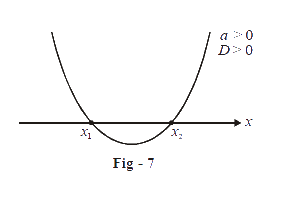The roots are given by $${x_1}\,\,{\rm{and}}\,\,{x_2}$$.

For what values of x is $$f\left( x \right) > 0?$$

Obviously, for those values for which the graph of $$f\left( x \right)$$ lies above the x-axis. i.e.

$f\left( x \right) > 0\,\,{\rm{for}}\,x < {x_1}\,\,{\rm{and}}\,\,x > {x_2}$

Similarly

$f\left( x \right) < 0\,\,{\rm{for}}\,{x_1} < x\,\, < {x_2}$

Consider another example: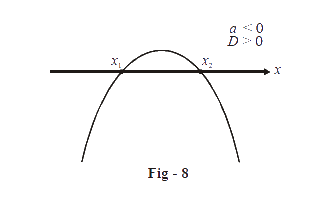We see from the graph that

\begin{align}f\left( x \right) > 0\,\,{\rm{for}}\;{x_1} < {x}\;< {x_2}\end{align}

and

\begin{align}f\left( x \right) < 0\;{\rm{for}}\,x < {x_1}\,\,{\rm{and}}\,\,x > {x_2}\end{align}

Finally, consider a third example: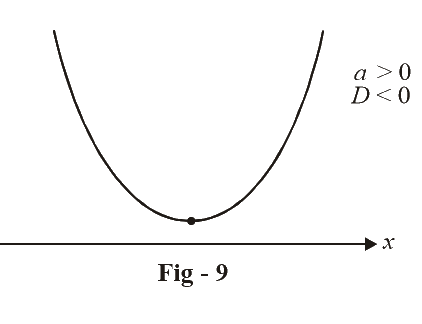The graph $$f\left( x \right)$$  for lies entirely above the x-axis.

Therefore

$$f\left( x \right) > 0$$ for all values of x

and

$$f\left( x \right) < 0$$ for no value of x

It is evident that quadratic inequalities can easily be solved by considering their corresponding graphs.

The results discussed above are summarized for convenience in the table below.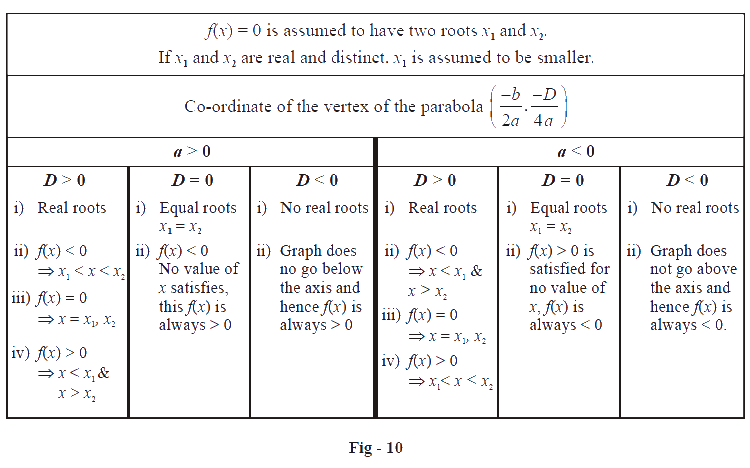Learn from the best math teachers and top your exams

• Live one on one classroom and doubt clearing
• Practice worksheets in and after class for conceptual clarity
• Personalized curriculum to keep up with school